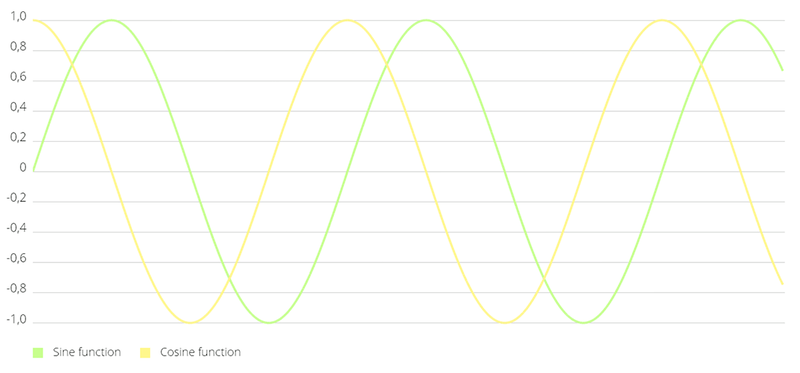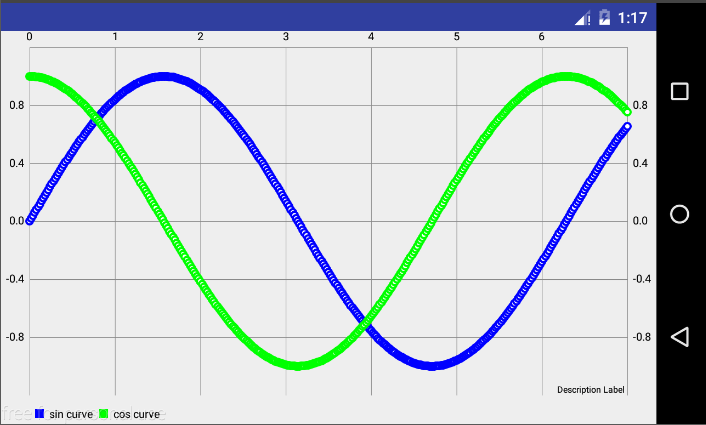Handling graph plots using MPAndroid chart in PSLab Android App

In PSLab Android App, we expose the Oscilloscope and Logic Analyzer functionality of PSLab hardware device. After reading data-points to plot, we need to show plot data on graphs for better understanding and visualisation. Sometimes we need to save graphs to show output/findings of the experiment. Hence we will be using MPAndroidChart library to plot and save graphs as it provides a nice and clean methods to do so.

dependencies {
compile 'com.github.PhilJay:MPAndroidChart:v3.0.2'
}

For chart view in your layout file, there are many available options like Line Chart, Bar Chart, Pie Chart, etc. For this post I am going to use Line Chart.

<com.github.mikephil.charting.charts.LineChart
android:id="@+id/lineChart"
android:layout_width="match_parent"
android:layout_height="match_parent" />

Take a reference to LineChart of layout file in your Activity/Fragment

LineChart lineChart = (LineChart) findViewById(R.id.chart);// Activity
LineChart lineChart = (LineChart) view.findViewById(R.id.chart);// Fragment

Now we add dataset to LineChart of layout file, I am going to add data for two curves sine and cosine function to plot sine and cosine wave on LineChart. We create two different LineDataSet one for the sine curve entries and other for the cosine curve entries.

List <Entry> sinEntries = new ArrayList<>(); // List to store data-points of sine curve
List <Entry> cosEntries = new ArrayList<>(); // List to store data-points of cosine curve

// Obtaining data points by using Math.sin and Math.cos functions
for( float i = 0; i < 7f; i += 0.02f ){
}

List<ILineDataSet> dataSets = new ArrayList<>(); // for adding multiple plots

LineDataSet sinSet = new LineDataSet(sinEntries,"sin curve");
LineDataSet cosSet = new LineDataSet(cosEntries,"cos curve");

// Adding colors to different plots
cosSet.setColor(Color.GREEN);
cosSet.setCircleColor(Color.GREEN);
sinSet.setColor(Color.BLUE);
sinSet.setCircleColor(Color.BLUE);

// Adding each plot data to a List

// Setting datapoints and invalidating chart to update with data points
lineChart.setData(new LineData(dataSets));
lineChart.invalidate();

After adding datasets to chart and invalidating it, chart is refreshed with the data points which were added in dataset.

After plotting graph output would look like the image below:You can change the dataset and invalidate chart to update it with latest dataset.

To save graph plot, make sure you have permission to write to external storage, if not add it into your manifest file

<manifest ...>
<uses-permission android:name="android.permission.WRITE_EXTERNAL_STORAGE" />
...
</manifest>

To save the photo of chart into Gallery:

lineChart.saveToGallery("title");

To save a some specific location:

lineChart.saveToPath("title", "Location on SD Card");

If you want to do some resizing in chart or save two three charts in a single image, you can do so by taking out the Bitmaps and processing them to meet your requirements:

lineChart.getChartBitmap();